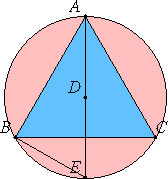# Proposition 12

If an equilateral triangle is inscribed in a circle, then the square on the side of the triangle is triple the square on the radius of the circle.

Let ABC be a circle, and let the equilateral triangle ABC be inscribed in it.

I say that the square on one side of the triangle ABC is triple the square on the radius of the circle.III.1

Take the center D of the circle ABC, join AD and carry it through to E, and join BE.

IV.15,Cor.

Then, since the triangle ABC is equilateral, therefore the circumference BEC is a third part of the circumference of the circle ABC. Therefore the circumference BE is a sixth part of the circumference of the circle. Therefore the straight line BE belongs to a hexagon. Therefore it equals the radius DE.

And, since AE is double DE, therefore the square on AE is quadruple the square on ED, that is, of the square on BE.

But the square on AE equals the sum of the squares on AB and BE. Therefore the sum of the squares on AB and BE is quadruple the square on BE.

Therefore, taken separately, the square on AB is triple the square on BE. But BE equals DE, therefore the square on AB is triple the square on DE.

Therefore the square on the side of the triangle is triple the square on the radius.

Therefore, if an equilateral triangle is inscribed in a circle, then the square on the side of the triangle is triple the square on the radius of the circle.

Q.E.D.

## Guide

#### Use of this proposition

This result is needed in proposition XIII.13 coming next to show that the construction given there produces a regular tetrahedron.